# Problem 52973. Easy Sequences 45: Second Derivative of Inverse Polynomial Function

The inverse of a function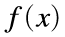, is the function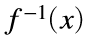, that reverses f. That means that if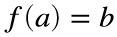, then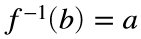. For example, the function to convert celsius temperature to fahrenheit is: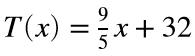, the inverse function (convert from fahrenheit to celsius) is: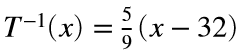. So that,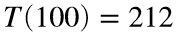and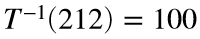.
Given a polynomial function P (presented as vector of numbers), and a value n, if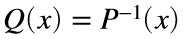, write a program that evaluates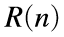, where.
For example, if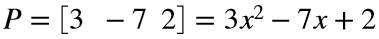, and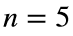, thenand. Therefore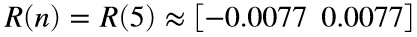.
NOTE: It is possible forto return some complex numbers. We are interested only with real values, so in cases where there are no real, please output an empty vector. Also please round-off your output to 4 decimal places, and sorted in ascending order.

### Solution Stats

35.71% Correct | 64.29% Incorrect
Last Solution submitted on Mar 19, 2023

### Community Treasure Hunt

Find the treasures in MATLAB Central and discover how the community can help you!

Start Hunting!